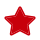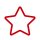# 数学运算函数

#### 关系运算符

A = B

BOOLEAN

A <> B

BOOLEAN

A < B

BOOLEAN

A <= B

BOOLEAN

A > B

BOOLEAN

A >= B

BOOLEAN

A IS NULL

BOOLEAN

A IS NOT NULL

BOOLEAN

A IS DISTINCT FROM B

BOOLEAN

A IS NOT DISTINCT FROM B

BOOLEAN

A BETWEEN [ASYMMETRIC | SYMMETRIC] B AND C

BOOLEAN

• ASYMMETRIC：表示B和C位置相关。

例如：A BETWEEN ASYMMETRIC B AND C 等价于 (A BETWEEN B AND C)。

• SYMMETRIC：表示B和C位置不相关。

例如：A BETWEEN SYMMETRIC B AND C 等价于 (A BETWEEN B AND C) OR (A BETWEEN C AND B)。

A NOT BETWEEN B [ASYMMETRIC | SYMMETRIC]AND C

BOOLEAN

• ASYMMETRIC：表示B和C位置相关。

例如：A NOT BETWEEN ASYMMETRIC B AND C 等价于 (A NOT BETWEEN B AND C)。

• SYMMETRIC：表示B和C位置不相关。

例如：A NOT BETWEEN SYMMETRIC B AND C 等价于 (A NOT BETWEEN B AND C) OR (A NOT BETWEEN C AND B)。

A LIKE B [ ESCAPE C ]

BOOLEAN

A NOT LIKE B [ ESCAPE C ]

BOOLEAN

A SIMILAR TO B [ ESCAPE C ]

BOOLEAN

A NOT SIMILAR TO B [ ESCAPE C ]

BOOLEAN

value IN (value [, value]* )

BOOLEAN

value NOT IN (value [, value]* )

BOOLEAN

EXISTS (sub-query)

BOOLEAN

value IN (sub-query)

BOOLEAN

value NOT IN (sub-query)

BOOLEAN

• double、real和float值存在一定的精度差。且我们不建议直接使用等号“=”对两个double类型数据进行比较。用户可以使用两个double类型相减，而后取绝对值的方式判断。当绝对值足够小时，认为两个double数值相等，例如:
`abs(0.9999999999 - 1.0000000000) < 0.000000001 //0.9999999999和1.0000000000为10位精度，而0.000000001为9位精度，此时可以认为0.9999999999和1.0000000000相等。`
• 数值类型可与字符串类型进行比较。做大小(>,<,>=,<=)比较时，会默认将字符串转换为数值类型，因此不支持字符串内有除数字字符之外的字符。
• 字符串之间可以进行比较。

A OR B

BOOLEAN

A AND B

BOOLEAN

NOT A

BOOLEAN

A IS FALSE

BOOLEAN

A IS NOT FALSE

BOOLEAN

A IS TRUE

BOOLEAN

A IS NOT TRUE

BOOLEAN

A IS UNKNOWN

BOOLEAN

A IS NOT UNKNOWN

BOOLEAN

#### 算术运算符

+ numeric

- numeric

A + B

A和B相加。结果数据类型与操作数据类型相关，例如一个整数类型数据加上一个浮点类型数据，结果数值为浮点类型数据。

A - B

A和B相减。结果数据类型与操作数据类型相关。

A * B

A和B相乘。结果数据类型与操作数据类型相关。

A / B

A和B相除。结果是一个double（双精度）类型的数值。

POWER(A, B)

ABS(numeric)

MOD(A, B)

SQRT(A)

LN(A)

LOG10(A)

LOG2(A)

LOG(B)

LOG(A, B)

B必须大于0，且A必须大于1。

EXP(A)

CEIL(A)

CEILING(A)

FLOOR(A)

SIN(A)

COS(A)

TAN(A)

COT(A)

ASIN(A)

ACOS(A)

ATAN(A)

ATAN2(A, B)

COSH(A)

DEGREES(A)

SIGN(A)

ROUND(A, d)

PI

E()

RAND()

RAND(A)

RAND_INTEGER(A)

RAND_INTEGER(A, B)

UUID()

BIN(A)

HEX(A)

HEX(B)

TRUNCATE(A, d)

truncate(42.345) = 42.000

PI()

# 相关产品